# 資料科學在文字分析中的應用 ：中英文 NLP（上）

《後疫情時代，資料科學賦能旅遊行業服務質量提升》這篇博文中，我們介紹了貓途鷹文字分析專案的背景和解決方案，並展示了最終的分析結果。接下來，對於中英文 NLP 感興趣的讀者，我們會為大家詳細講解資料採集、資料入庫、資料清理和資料建模步驟中涉及的原理和程式碼實現。由於篇幅的限制，上篇會重點講解資料採集、資料入庫和資料清理這三個步驟，下篇則會講解資料建模的完整流程。

## 資料採集

### 1.  抓取工具分析

Beautiful Soup

Beautiful Soup 是幾種工具中最容易上手的網頁抓取庫，它可以快速幫助開發者從 HTML 或 XML 格式的檔案中獲取資料。在這個過程中，Beautiful Soup 會一定程度上讀取這類檔案的資料結構，並在此基礎上提供許多與查詢和獲取資料內容相關的方程。除此之外，Beautiful Soup 完善、易於理解的文件和活躍的社群使得開發者不僅可以快速上手，也能快速精通，並靈活運用於開發者自己的應用當中。

Scrapy

Scrapy 是非常受歡迎的開源網頁抓取庫之一，它最突出的特性是抓取速度快，又因為它基於 Twisted 非同步網路框架，使用者傳送的請求是以無阻塞機制傳送給伺服器的，比阻塞機制更靈活，也更節省資源。因此，Scrapy 擁有了以下這些特性：

• 對於 HTML 型別網頁，使用XPath或者CSS表述獲取資料的支援
• 可運行於多種環境，不僅僅侷限於 Python。Linux、Windows、Mac 等系統都可以使用 Scrapy 庫。
• 擴充套件性強
• 速度和效率較高
• 需要的記憶體、CPU 資源較少

Selenium

Selenium 的起源是為了測試網頁應用程式而開發的，它獲取網頁內容的方式與其他庫截然不同。Selenium 在結構設計上是通過自動化網頁操作來獲取網頁返回的結果，和 Java 的相容性很好，也可以輕鬆應對 AJAX 和 PJAX 請求。和 Beautiful Soup 相似，Selenium 的上手相對簡單，但與其他庫相比，它最大的優勢是可以處理在網頁抓取過程中出現的需要文字輸入才能獲取資訊、或者是彈出頁面等這種需要使用者在瀏覽器中有介入動作的情況。這樣的特性使得開發者對網頁抓取的步驟更加靈活，Selenium 也因此成為了最流行的網頁抓取庫之一。

### 2.  網頁資料和結構的初步瞭解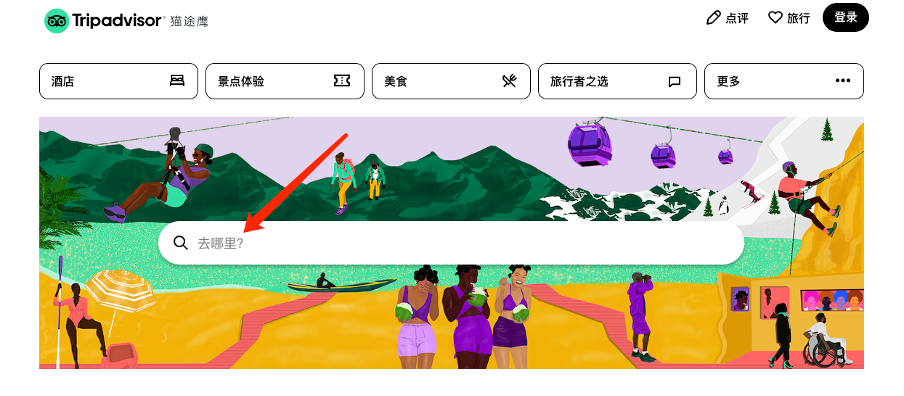• 貓途鷹的搜尋引擎本身會對景點名稱和搜尋輸入進行比較，通過自己內部的邏輯將符合條件的景點排名靠前
• 我們可以在結果出現後使用省份、城市等資訊篩選得到目標景點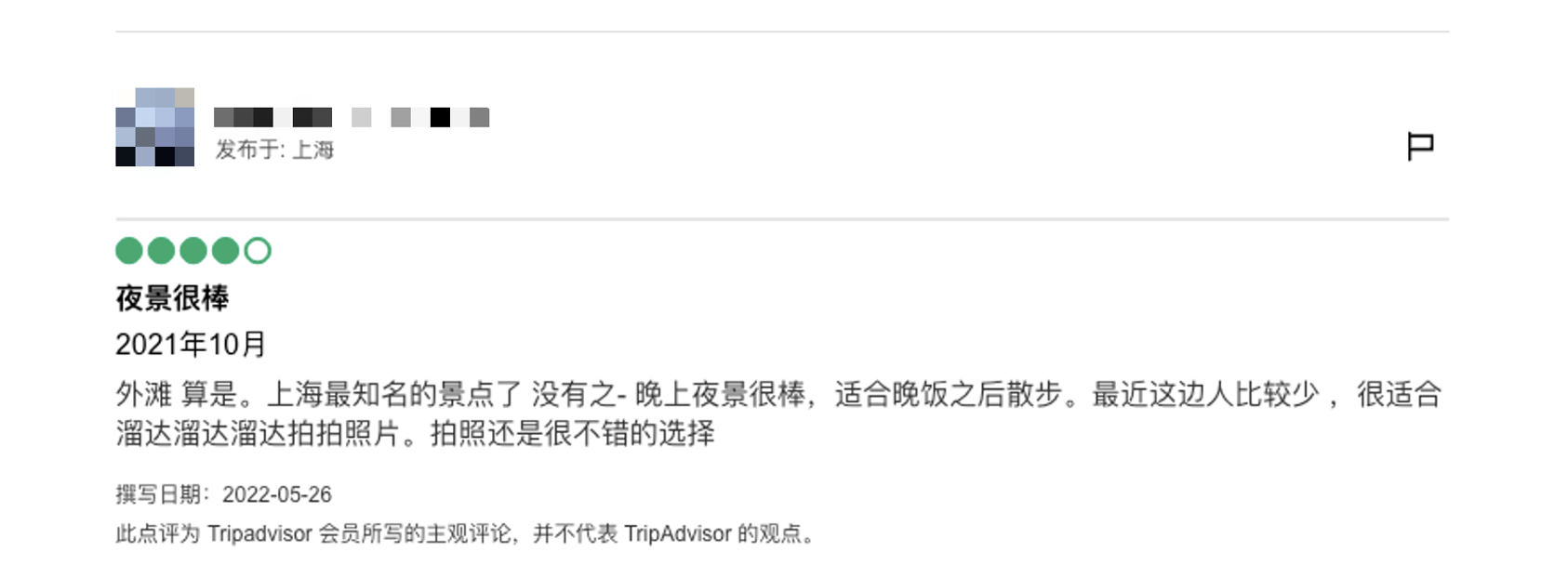• 使用者
• 使用者所在地
• 評分
• 點評標題
• 到訪日期
• 旅行型別
• 詳細點評
• 撰寫日期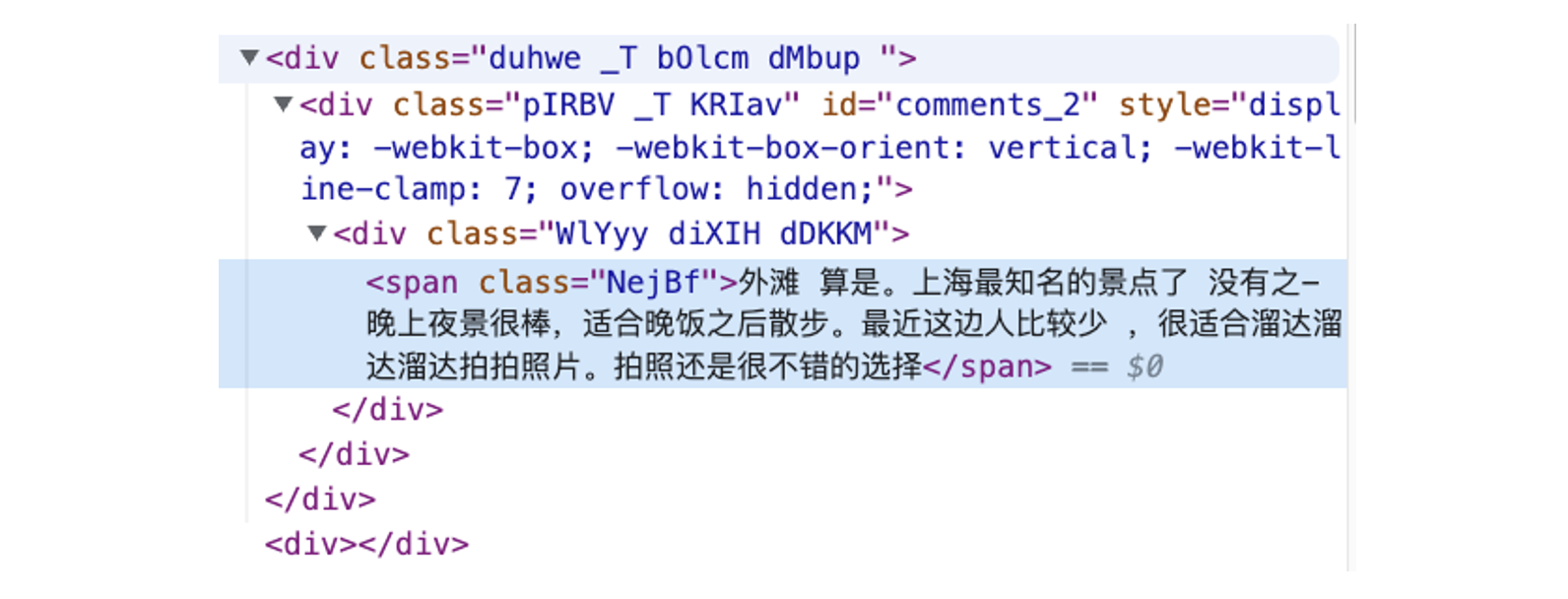• 第一步：傳送請求，使用 Selenium 操作瀏覽器找到指定景點的評論頁面
• 第二步：進入評論頁面，抓取評論資料

### 3.  獲取評論資料

```from selenium import webdriver
import chromedriver_binary
from webdriver_manager.chrome import ChromeDriverManager
from selenium.webdriver.common.by import By
from selenium.webdriver.common.keys import Keys
import time
import datetime
import re
import pandas as pd

```#utility.py
import time
import configparser
def print_log_message(app_name, procedure, message):
ts = time.localtime()
print(time.strftime("%Y-%m-%d %H:%M:%S", ts) + " **" + app_name + "** " + procedure + ":", message)
return
config = configparser.ConfigParser()
var_value = config.get(section, var)
return var_value```

```# Initiate web session
chrome_options = webdriver.ChromeOptions()
wd = webdriver.Chrome(ChromeDriverManager().install(),chrome_options=chrome_options)
wd.get(self.web_url)
wd.implicitly_wait(5)
review_results = {}```

```location_name = '外灘'
city = '上海'
state = '上海' ```
```# Find search box
wd.find_element(By.CSS_SELECTOR, '.weiIG.Z0.Wh.fRhqZ>div>form>input').click()```
```# Enter location name
wd.find_element(By.XPATH, '//input[@placeholder="去哪裡？"]').send_keys(f'{location_name}')
wd.find_element(By.XPATH, '//input[@placeholder="去哪裡？"]').send_keys(Keys.ENTER)```
```# Find the right location with city + province info
element = wd.find_element(By.XPATH,
f'//*[@class="address-text" and contains(text(), "{city}") and contains(text(), "{state}")]')
element.click()```

`comment_section = wd.find_element(By.XPATH, '//*[@data-automation="WebPresentation_PoiReviewsAndQAWeb"]')`
```# user id
user_elements = comment_section.find_elements(By.XPATH, '//div[@class="ffbzW _c"]/div/div/div/span[@class="WlYyy cPsXC dTqpp"]')
user_list = [x.text for x in user_elements]```

```# location
loca_elements = comment_section.find_elements(By.XPATH,
'//div[@class="ffbzW _c"]/div/div/div/div/div[@class="WlYyy diXIH bQCoY"]')
loca_list = [x.text[5:] for x in loca_elements]```
```# trip type
trips_element = comment_section.find_elements(By.XPATH, '//*[@class="eRduX"]')
trip_types = [self.separate_trip_type(x.text) for x in trips_element]```

```# comment date
```# drop out the first element

```# rating
rating_element = comment_section.find_elements(By.XPATH,
'//div[@class="dHjBB"]/div/span/div/div[@style="display: block;"]')
rating_list = []
for rating_code in rating_element:
code_string = rating_code.get_attribute('innerHTML')
s_ind = code_string.find(" bubble_")
rating_score = code_string[s_ind + len(" bubble_"):s_ind + len(" bubble_") + 1]
rating_list.append(rating_score)```
```# comments title
'//*[@class="WlYyy cPsXC bLFSo cspKb dTqpp"]')
```# comments content
'//*[@data-automation="WebPresentation_PoiReviewsAndQAWeb"]'
).find_elements(By.XPATH, '//*[@class="duhwe _T bOlcm dMbup "]')

```# if review contains pictures
pic_sections = comment_section.find_elements(By.XPATH,
'//div[@class="ffbzW _c"]/div[@class="hotels-community-tab-common-Card__card--ihfZB hotels-community-tab-common-Card__section--4r93H comment-item"]')
pic_list = []
for r in pic_sections:
if 'background-image' in r.get_attribute('innerHTML'):
pic_list.append(1)
else:
pic_list.append(0)```

```#引入之前定義的Python Class：
from data_processor import WebScrapper
scrapper = WebScrapper()```
```#執行網頁抓取方程抓取中文語料：
trip_review_data = scrapper.trip_advisor_zh_scrapper_runner(location, location_city, location_state, page_n=int(n_pages))```

## 資料入庫

```from sqlalchemy import create_engine
import psycopg2

`data.to_sql(table_name, engine, if_exists=‘replace’, index=False)`

data 是我們需要入庫的 Pandas DataFrame 資料，table_name 是表名，engine 是我們之前定義的 SQLAlchemy 引擎, if_exists=‘replace’ 和 index=False 則是 Pandas to_sql() 方程的選項。這裡選項的含義是，如果表已存在則用現有資料替代已有資料，並且在入庫過程中，我們不需要考慮索引。

## 資料清洗

• 使用者資訊（如所在地等）
• 評論資訊（如是否包含圖片資訊等）
• 評論語料

```import numpy as np
import pandas as pd
import psycopg2
from sqlalchemy import create_engine
import langid
import re
import emoji
from sklearn.preprocessing import MultiLabelBinarizer
import demoji
import random
from random import sample
import itertools
from collections import Counter
import matplotlib.pyplot as plt```

```df = pd.read_sql('SELECT * FROM "上海_上海_外灘_source_review"', engine)
df.shape
```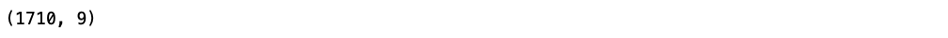```df = pd.read_sql('SELECT * FROM "Shanghai_Shanghai_The Bund (Wai Tan)_source_review_EN"', engine)
df.shape
```### 1.  資料型別處理

```df['comment_date'] = pd.to_datetime(df['comment_date'])
df['rating'] = df['rating'].astype(str)
df['comment_year'] = df['comment_date'].dt.year
df['comment_month'] = df['comment_date'].dt.month```

### 2.  瞭解資料狀況

`df.isnull().sum()`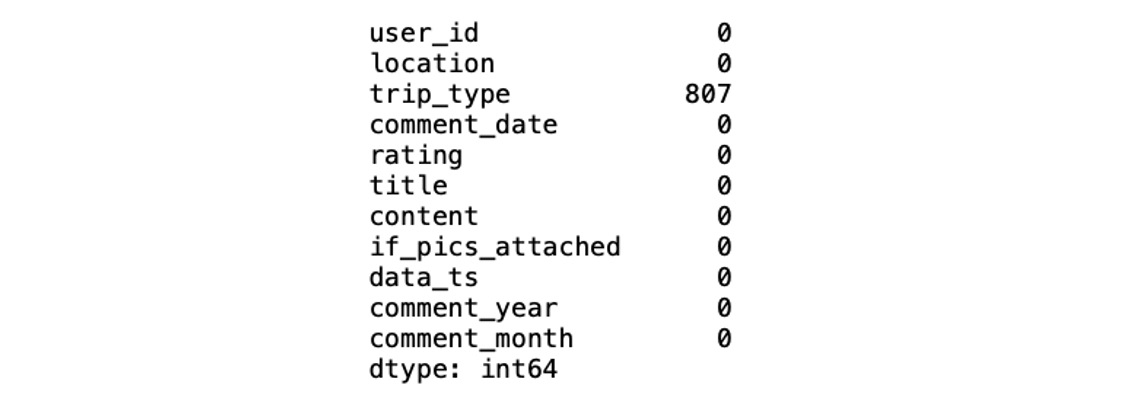### 3.  處理旅行型別空值

`df.groupby(['trip_type']).size()`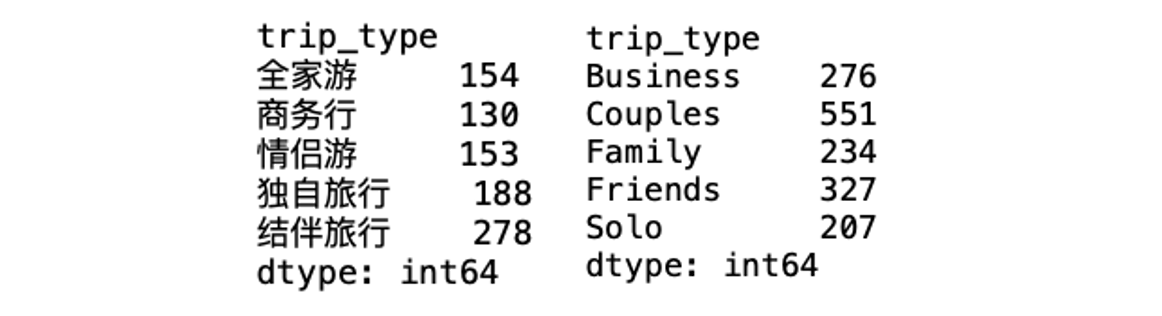`df['trip_type'] = df['trip_type'].fillna('未知')`

`df['trip_type'] = df['trip_type'].fillna('NA')`

```zh_trip_type = ['全家遊', '商務行', '情侶遊', '獨自旅行', '結伴旅行', '未知']
en_trip_type = ['Family', 'Business', 'Couples', 'Solo', 'Friends', 'NA']
trip_type_df = pd.DataFrame({'zh_type':zh_trip_type, 'en_type':en_trip_type})```

`trip_type_df.to_sql("tripadvisor_TripType_lookup", engine, if_exists="replace", index=False)`

### 4.  處理英文評論資料中使用者所在地資訊

• 如果地區資訊用逗號隔開，前一個詞為城市，後一個詞為國家/省份
• 如果沒有逗號，則預設該資訊為國家資訊

• 將縮寫的國家/省份提取出來並單獨處理（以美國為主，使用者在填寫地區資訊時只填寫州名）
• 檢視除縮寫以外的國家資訊，如國家名稱未出現在國家列表裡，則認為是城市資訊
• 國家欄位中出現的城市名錯填（如大型城市）和拼寫錯誤問題，則手動修改處理

```country_file = open("countries.txt", "r")
country_list = country_data.split("\n")
countries_lower = [x.lower() for x in country_list]

```def formating_country_info(s_input):
if s_input is None: #若字串輸入為空值，返回空值
return None
if s_input.strip().lower() in countries_lower: #若字串輸入在國家列表中，返回國家名
c_index = countries_lower.index(s_input.strip().lower())
return country_list[c_index]
else:
if len(s_input) == 2: #若輸入為縮寫，在美國州名、墨西哥省名和英國縮寫中查詢，若可以找到，返回對應國家名稱
if s_input.strip().upper() in state_code["code"].to_list():
return "United States"
elif s_input.strip().upper() == "UK":
return "United Kingdom"
elif s_input.strip().upper() in ("RJ", "GO", "CE"):
return "Mexico"
elif s_input.strip().upper() in ("SP", "SG"):
return "Singapore"
else:
# could not detect country info
return None
else: #其他情況，需要手動修改國家名稱
if s_input.strip().lower() == "caior":
return "Egypt"
else:
return None```

`df["location_country"] = df["location_country"].apply(formating_country_info)`

`df["location_country"].isnull().sum()`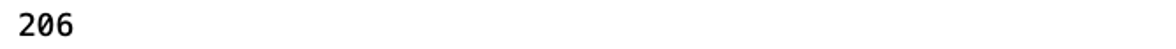```def check_if_country_info(city_list):
clean_list = []
country_fill_list = []
for city in city_list:
if city is None:
clean_list.append(None)
country_fill_list.append(None)
elif city.strip().lower() in countries_lower: #如城市變數中出現的是國家名，記錄國家名稱
c_index = countries_lower.index(city.strip().lower())
country_name = country_list[c_index]
if country_name == "Singapore": #如城市名為新加坡，保留城市名，如不是則將原先的城市名轉換為空值
clean_list.append(country_name)
else:
clean_list.append(None)
country_fill_list.append(country_name)
else:
# format city string
city_name = city.strip().lower().capitalize()
clean_list.append(city_name)
country_fill_list.append(None)
return clean_list, country_fill_list```

`city_list, country_fillin = check_if_country_info(df["location_city"].to_list())`

`df["country_fill_temp"] = country_fillin`

```df["location_city"] = city_list
df["location_country"] = df["location_country"].fillna(df["country_fill_temp"])
df = df.drop(columns=["country_fill_temp"])```